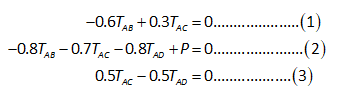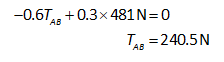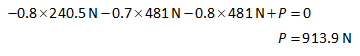# Three cables are used to tether a balloon as shown. Determine the vertical force P exerted by the balloon at A knowing that the tension in cable AD is 481 N.

Question-AnswerCategory: Engineering MechanicsThree cables are used to tether a balloon as shown. Determine the vertical force P exerted by the balloon at A knowing that the tension in cable AD is 481 N.

### Three cables are used to tether a balloon as shown. Determine the vertical force P exerted by the balloon at A knowing that the tension in cable AD is 481 N.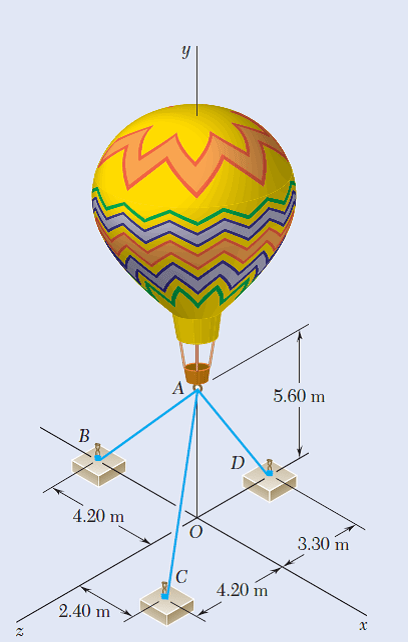Step 1

Let us consider that
The tension along AB is TAB.
The tension along AC is TAC.
Express the forces in unit vectors:Determine the torque vectors for the following:Step 2

Apply equilibrium conditions:TAB is the torque along AB.
TAC is the torque along AC.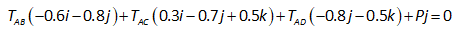Compare the coefficients with the zero vector: